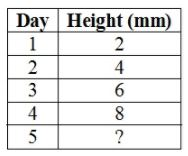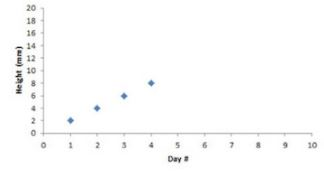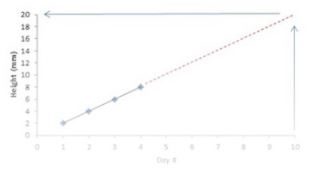Courses

# Extrapolation - Interpolation and Extrapolation, Business Mathematics and Statistics B Com Notes | EduRev

Created by: Arshit Thakur

## B Com : Extrapolation - Interpolation and Extrapolation, Business Mathematics and Statistics B Com Notes | EduRev

The document Extrapolation - Interpolation and Extrapolation, Business Mathematics and Statistics B Com Notes | EduRev is a part of the B Com Course Business Mathematics and Statistics.
All you need of B Com at this link: B Com

Extrapolation is a useful statistical tool used to estimate values that go beyond a set of given data or observations. In this lesson, you will learn how to estimate or predict values using this tool.

Definition and Use of Extrapolation

Extrapolation is the process of finding a value outside a data set. It could even be said that it helps predict the future! To help us remember what it means, we should think of the part of the word 'extra' as meaning 'more' data than what we originally had. This tool is not only useful in statistics but also useful in science, business, and anytime there is a need to predict values in the future beyond the range we have measured. There are several methods for extrapolation, but in this lesson we will focus on linear extrapolation, which is using a linear equation to find a value outside a data set.

Example 1

Let's try a basic extrapolation by finding values in a numerical sequence. When using extrapolation, we look for the relationship between the given values. So, let's look at the following numerical sequence. What is the relationship between the values in the sequence?

2,4,6,8, ?

Pretty easy, right? The numbers in the sequence are increasing by 2. Now, by using extrapolation we can predict the fifth term in each sequence. The fifth term of the sequence is 10. However, extrapolation goes beyond estimating future values in numerical sequences, as we will see in the next example.

Example 2

My friend Mary planted a bean plant, and she has been measuring and keeping track of its growth for the past four days. Based on her observations, she wants to estimate how tall her plant will be on the 5th day.

Her chart of observations looks like this:Based on Mary's chart, it is not too difficult to predict that the plant will be 10 mm tall on the fifth day. But, what if Mary wanted to predict the plant's height on the tenth day? In that case, extrapolating from a graph would come in handy. To illustrate this, let's plot her observations on a graph. When we plot the data, we realize there is a linear relationship between the number of days and the growth of the plant.On the graph, we can also see that there are data points for four days of observation. However, Mary wants to know how tall her plant will be on the tenth day. This would not be too difficult to do using extrapolation. We just need to draw a line through the data points and then extend the line past the tenth day mark.

If we take a look at the next graph, we can see the extended line and with that, we can estimate that the height of the plant would be 20 mm on the tenth day.Offer running on EduRev: Apply code STAYHOME200 to get INR 200 off on our premium plan EduRev Infinity!

122 videos|142 docs

,

,

,

,

,

,

,

,

,

,

,

,

,

,

,

,

,

,

,

,

,

,

,

,

;